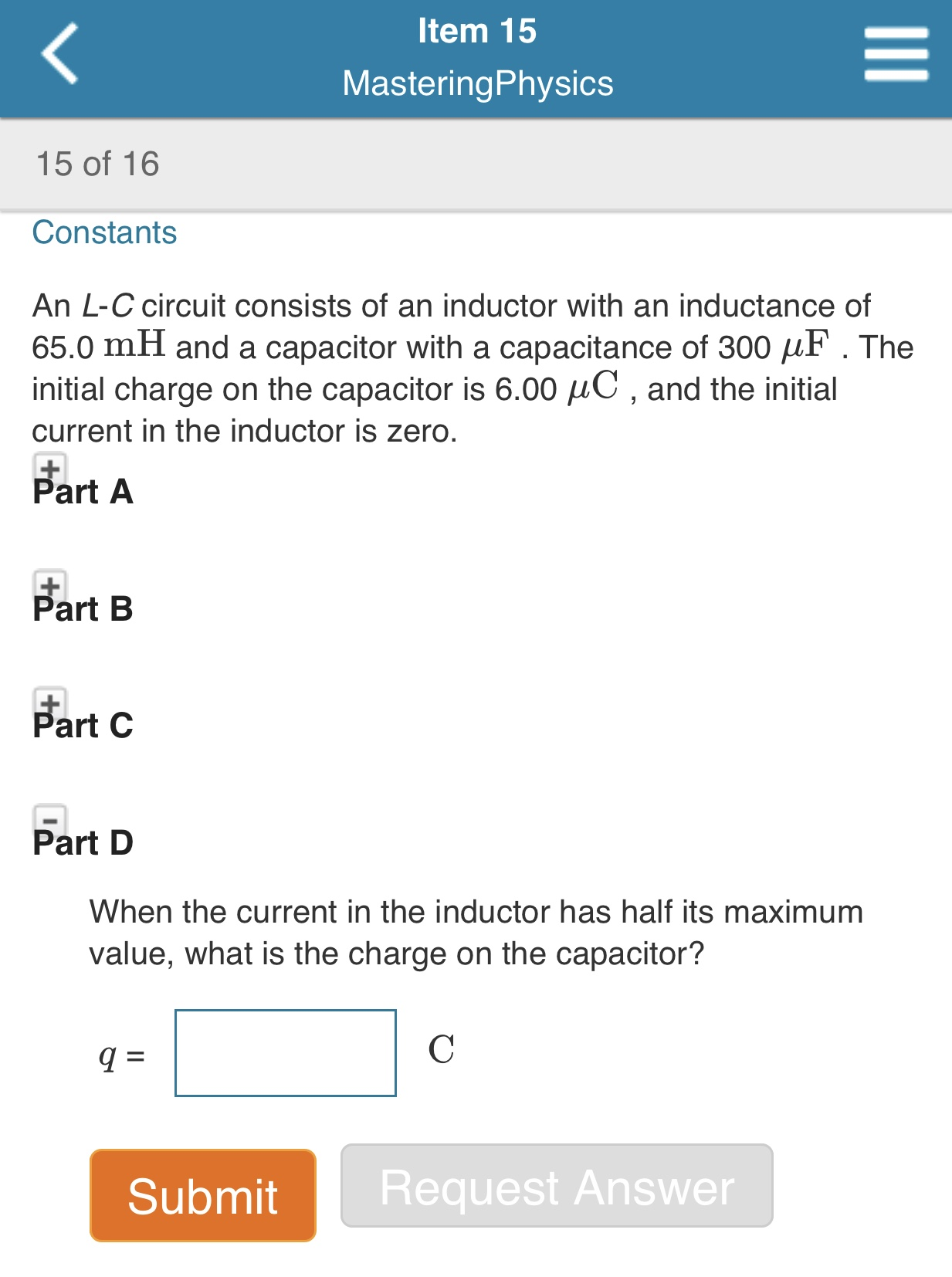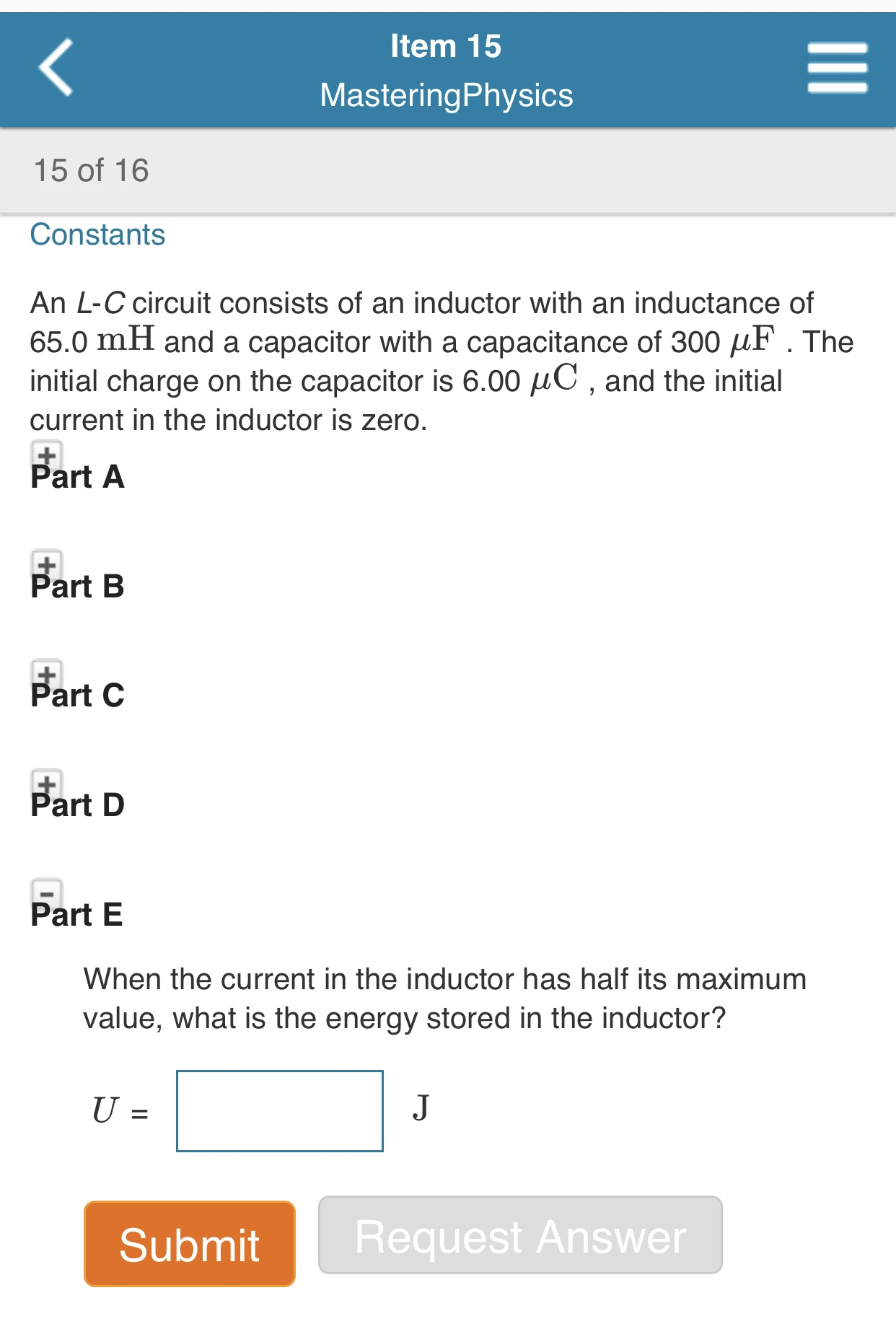# Item 15 MasteringPhysics 15 of 16 Constants An L-C circuit consists of an inductor with an inductance of 65.0 mH and a capacitor with a capacitance of 300 HF. The initial charge on the capacitor is 6.00 C , and the initial current in the inductor is zero. Part A + Part B Part C Part D When the current in the inductor has half its maximum value, what is the charge on the capacitor? Request Answer Submit II Item 15 MasteringPhysics 15 of 16 Constants An L-C circuit consists of an inductor with an inductance of 65.0 mH and a capacitor with a capacitance of 300 F. The initial charge on the capacitor is 6.00 HC, and the initial current in the inductor is zero. Part A Part B Part C Part D Part E When the current in the inductor has half its maximum value, what is the energy stored in the inductor? J U = 1 Request Answer Submit II

Questionhelp_outlineImage TranscriptioncloseItem 15 MasteringPhysics 15 of 16 Constants An L-C circuit consists of an inductor with an inductance of 65.0 mH and a capacitor with a capacitance of 300 HF. The initial charge on the capacitor is 6.00 C , and the initial current in the inductor is zero. Part A + Part B Part C Part D When the current in the inductor has half its maximum value, what is the charge on the capacitor? Request Answer Submit II fullscreenhelp_outlineImage TranscriptioncloseItem 15 MasteringPhysics 15 of 16 Constants An L-C circuit consists of an inductor with an inductance of 65.0 mH and a capacitor with a capacitance of 300 F. The initial charge on the capacitor is 6.00 HC, and the initial current in the inductor is zero. Part A Part B Part C Part D Part E When the current in the inductor has half its maximum value, what is the energy stored in the inductor? J U = 1 Request Answer Submit II fullscreen